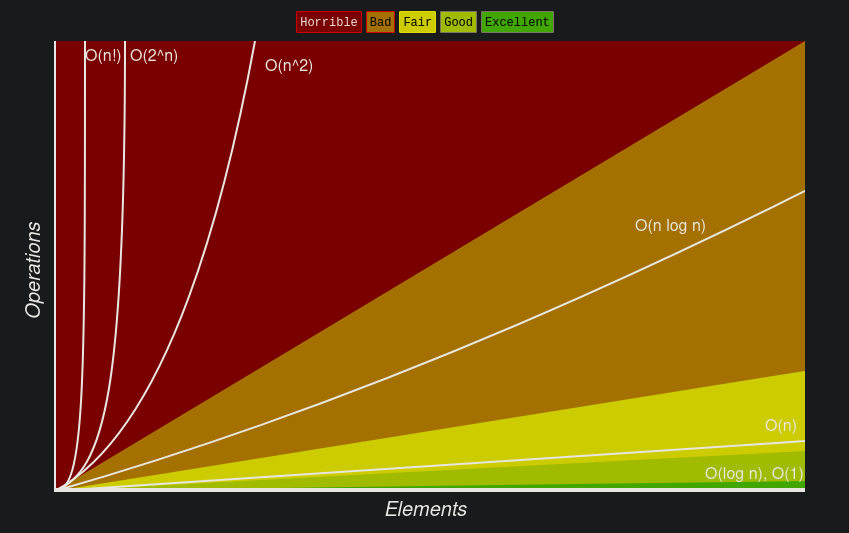# Languages

## Search, Data Structures and Array Sorting

Time Complexity (Average, Worst), Space (Worst)search (A)search (W)space (W)
Binary Search (C, Python)O(log(n))O(log(n))O(1)
Time Complexity (Average, Worst)search (A)insert (A)delete (A)search (W)insert (W)delete (W)
Array (C, Python)O(n)O(n)O(n)O(n)O(n)O(n)
Stack (C, Python)O(n)O(1)O(1)O(n)O(1)O(1)
Queue (C, Python)O(n)O(1)O(1)O(n)O(1)O(1)
Hash Table (C, Python)O(1)O(1)O(1)O(n)O(n)O(n)
Binary Heap (C, Python, notes)max,min O(1), O(n)O(log(n))O(log(n))O(?)O(log(n))O(log(n))
Tree
Graph
Binary Search Tree (C, Python)O(log(n))O(log(n))O(log(n))O(n)O(n)O(n)
B-Tree (C, Python)O(log(n))O(log(n))O(log(n))O(log(n))O(log(n))O(log(n))

Notes:

• access is ommitted because it is generally the same complexity than search (except for some case such as array)
• https://en.wikipedia.org/wiki/List_of_data_structures
Complexity (Time, Space)best (T)average (T)worst (T)worst (S)
Timsort (C, Python)O(n)O(n log(n))O(n log(n))O(n)
Heapsort (C, Python)O(n log(n))O(n log(n))O(n log(n))O(1)
Mergesort (C, Python)O(n log(n))O(n log(n))O(n log(n))O(n)
Quicksort (C, Python)O(n log(n))O(n log(n))O(n^2)O(log(n))
Bubblesort (C, Python)O(n)O(n^2)O(n^2)O(1)Source for complexity

Python Data Structure Complexity

## Resources

• geekforgeeks : Data Structures, Languages, Exercices and interviews

## Design Patterns - GoF

Gang of Four, are the initial people who wrote a famous book

gof.pdf